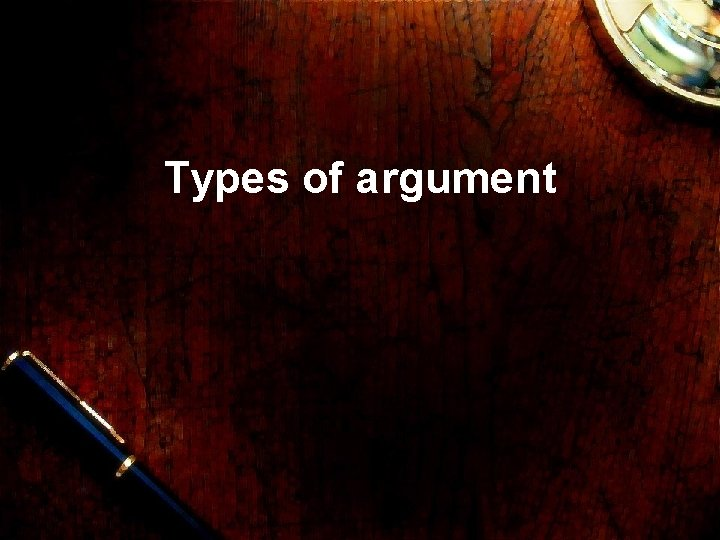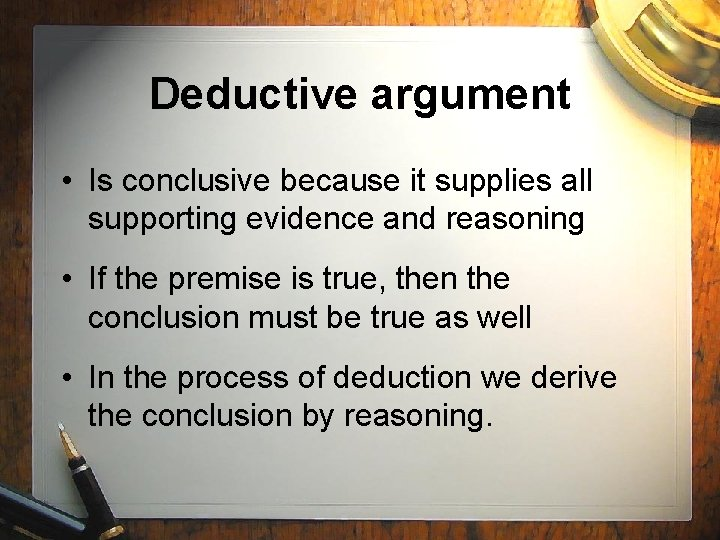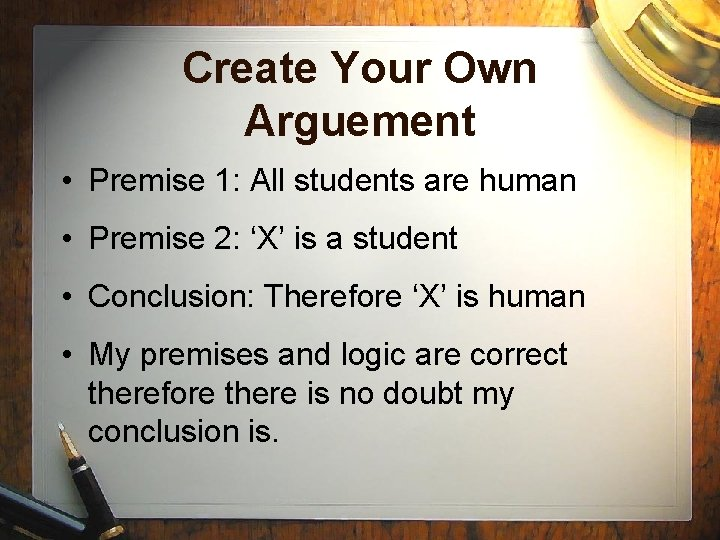# Types of argument There are two types of

• Slides: 10Types of argumentThere are two types of argument • Inductive • DeductiveDeductive argument • Is conclusive because it supplies all supporting evidence and reasoning • If the premise is true, then the conclusion must be true as well • In the process of deduction we derive the conclusion by reasoning.Example of a deductive argument • Premise 1: All students are human • Premise 2: ‘X’ is a student • Conclusion: Therefore ‘X’ is human • My premises and logic are correct therefore there is no doubt my conclusion is.Inductive arguments • Useful but limited • Cannot guarantee truth • Infer from the properties of a sample to the properties of a population as a whole.For example; • • I have a jar containing 1000 beans I draw out 100 50 are black and 50 are white Using inductive logic I can assume half (500) beans in the jar are blackCreate Your Own Arguement • Premise 1: All students are human • Premise 2: ‘X’ is a student • Conclusion: Therefore ‘X’ is human • My premises and logic are correct therefore there is no doubt my conclusion is.In inductive arguments • We generalise from a number (n) of cases that we know to be true • Infer that the same thing will remain true in all future cases • In inductive arguments the conclusion is based on two premises: – a generalisation from a few instances – the factInductive arguments are • Evaluated according to the degree to which the conclusion is probably true • Inductive argument is neither valid nor invalid.